Courses

Revision Notes - Equilibrium Notes | Study JEE Revision Notes - JEE

JEE: Revision Notes - Equilibrium Notes | Study JEE Revision Notes - JEE

The document Revision Notes - Equilibrium Notes | Study JEE Revision Notes - JEE is a part of the JEE Course JEE Revision Notes.
All you need of JEE at this link: JEE

Equilibrium

• Equilibrium is the state of a process in which the properties like temperature, pressure, and concentration etc of the system do not show any change with passage of time.
• In all processes which attain equilibrium, two opposing processes are involved.
• Equilibrium is attained when the rates of the two opposing processes become equal.
• If the opposing processes involve only physical changes, the equilibrium is called Physical Equilibrium.
• If the opposing processes are chemical reactions, the equilibrium is called Chemical Equilibrium.

Physical Equilibrium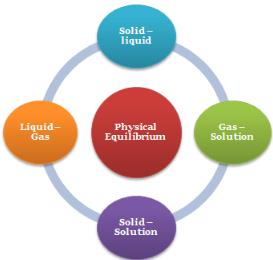• Solid – liquid Equilibrium: H2O(s) ⇌  H2O(l)
• Liquid – Gas Equilibrium: H2O(l) ⇌ H2O(g)
• Solid – Solution Equilibrium: Salt(Solid) ⇌ Salt(in solution)
• Gas –Solution equilibrium: CO2(g) ⇌ CO2(in solution)

Equilibrium in Chemical Process

• Reversible reaction:  A reaction in which not only the reactants react to form the products under certain conditions but also the products react to form reactants under the same conditions
Examples: 3Fe(s) + 4H2O(g) ⇌ Fe3O4(s) + 4H2(g)
• Irreversible reaction: A reaction cannot take place in the reverse direction, i.e. the products formed do not react to give back the reactants under the same condition.
Example: AgNO3(aq) + NaCl(aq) → AgCl(s) + NaNO3(g)
• Generally, a chemical equilibrium is represented as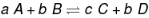Where A, B are reactants and C, D are products.

Note:
The double arrow between the left hand part and right hand part shows that changes are taking place in both the directions.
On the basis of extent of reaction, before equilibrium is attained chemical reactions may be classified into three categories.

• Those reactions which proceed to almost completion.
• Those reactions which proceed to almost only upto little extent.
• Those reactions which proceed to such an extent, that the concentrations of reactants and products at equilibrium are comparable.
• The equilibrium state is dynamic and not static in nature. A reaction is said to have attained equilibrium when the rate of forward reaction equals that of backward reaction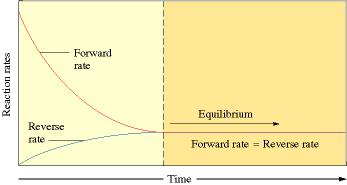• Homogeneous equilibrium: All the reactants and products of any reaction under equilibrium are in same physical state. Example:  N2(g) +  3H2(g) ⇌ 2NH3 (g)
• Heterogeneous equilibrium: Physical state of one or more of the reacting species may differ i.e. all the reactants and products are not in same physical state.Example
2NaHCO3(s) ⇌ Na2CO3(s) + CO2(g) + H2O(l)

Characteristics of Equilibrium State

• It can be attained only if the reversible reaction is carried out in closed vessel.
• It can be attained from either side of the reaction.
• A catalyst can hasten the approach of equilibrium but does not alter the state of equilibrium.
• It is dynamic in nature i.e. reaction does not stop but both forward and backward reactions take place at equal rate.
• Change of pressure, concentration or temperature favours one of the reactions (forward or backward) resulting in shift of equilibrium point in one direction.

Law of Mass Action & Equilibrium Constant
“The rate at which a substance reacts is directly proportional to its active mass and rate of a chemical reaction is directly proportional to product of active masses of reactants each raised to a power equal to corresponding stoichiometric coefficient appearing in the balanced chemical equation”.
Fore reaction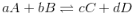Rate of reaction ∝ [A]a.[B]b
or rate of reaction = K[A]a[B]b
where K is rate constant or velocity constant of the reaction at that temperature.

Unit of rate constant (K)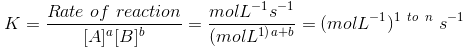(where n is order of reaction.)
For unit concentration of reactants rate of the reaction is equal to rate constant or specific reaction rate.

Note:
Active mass is the molar concentration of the reacting substances actually participating in the reaction.
Hence,
Active mass = number of moles/volume in litres
Active mass of solid is taken as unity.
Also, Active mass of reactant (a) = Conc. × activity coefficient
i.e. a = Molarity × f for dilute solution f = 1

Applying Law of mass action for general reversible reaction
aA + bB ⇌ cC + dD
Rate of forward reaction α [A]a[B]b
or Rf = Kf [A]b [B]b
Similarly for backward reaction
Rb = Kb[C]c [D]d
At equilibrium Kf[A]a[B]= Kb[C]c[D]d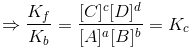The above equation is known as equilibrium equation and Kc is known as equilibrium constant. Equilibrium constant for the reverse reaction is the inverse of the equilibrium constant for the reaction in the forward direction.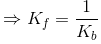Expression of KC
Let”a” moles of PCl5 be taken in a closed vessel of volume ‘V’ litre. Suppose “x” mole is dissociated at equilibrium.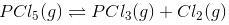Initial Conc: a,0,0
At. eqbm, a-x, x,x
Active mass (a-x)/V, x/V, x/V
According to law of mass action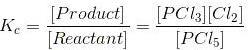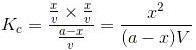Equilibrium Quotient or Mass Action Ratio:
Consider the equilibrium
aA +bB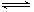cC +dD
At equilibrium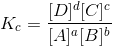When the reaction is not at equilibrium this ratio is called ‘QC’ i.e., QC is the general term used for the above given ratio at any instant of time. And at equilibrium QC becomes KC.

• If the reaction is at equilibrium, Q = Kc
• A net reaction proceeds from left to right (forward direction) if Q < KC.
• A net reaction proceeds from right to left (the reverse direction) if Q >Kc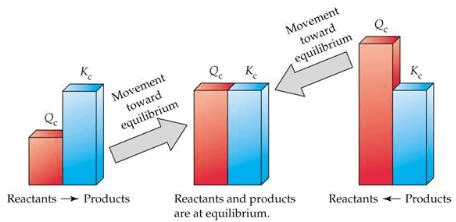The Le–Chatelier’s Principle:
Statement:
“When a chemical reaction at equilibrium is subjected to any stress, then the equilibrium shifts in that direction in which the effect of the stress is  reduced.”

Effect of Addition of Inert Gases:

• Addition of inert gas at constant volume: No effect on equilibrium.
• Addition of inert gas at constant pressure: Equilibrium shifts in a direction where there is increase in number of moles of gases.

Effect of Change in Temperature:

• In a system at equilibrium, both exothermic and endothermic reactions take place simultaneously.
• Increase in temperature would shift the equilibrium in the direction of endothermic reaction.
• Decrease in temperature would shift the equilibrium in the direction of exothermic reaction.

Effect of Change in Concentration:

• When the concentration of reactants increased, equilibrium shifts in forward direction.
• When the concentration of the products is increases, equilibrium shifts in backward direction.

Effect of Change in Pressure:

• Increase in pressure shifts the equilibrium in the direction of lesser number of gaseous molecules.
• Decrease in pressure shifts the equilibrium in the direction of larger number of gaseous molecules.

Effect of Catalyst:
Catalyst does not change the equilibrium.
Thermodynamics of chemical equilibrium:
ΔG = ΔG0 + 2.303 RT log Q
At equilibrium:
ΔG = ΔG0 + 2.303 RT log K = 0
or
ΔG= - 2.303 RT log K
Also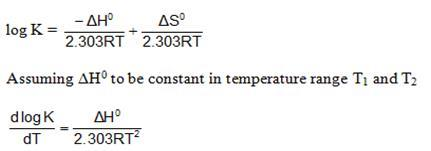Acids and Bases:
1. Arrhenius Definition:
a. Acids give H+ ions aqueous solutions.
b. Bases give OH- ions in aqueous solution.
2. Bronsted – Lowry Definition:
a. Acid is a proton donor .
b. Base is a proton acceptor.
3. Strong and Weak Acids:
a. Strong acid dissociate completely in aqueous solution.
Example: HCl
b. Weak acid dissociates partially in aqueous solution.
Examples: CH3COOH
Degree of dissociation (a) =(Ka/C)1/2
Where,
Ka = [CH3COO-][H+]/[CH3COOH]
And
C = Initial concentration of acid.
Also
[H+] = Ca = (Ka×C)1/2
c. Ionic Product of Water:
H2O  H+ + OH
K[H2O] = [H+] [OH] = 10-14

Common Ion effect:
The addition of an ion to equilibrium, having the same ion makes the equilibrium reaction move in a direction to consume that ion.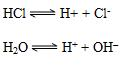This implies that water would dissociate less in the presence of HCl.

Hydrolysis of salts:
1. Salt of a Weak Acid and Strong Base:
[H+] = (Kw Ka / C )1/2
2. Salt of a Weak Base and Strong Acid
[H+] = (Kw / Kb C )1/2
3. Salt of a Weak Acid and Weak Base
[H+] = (Kw K/ Kb )1/2

• Buffer Solutions: Resists change in its pH when such a change is caused by the addition of a small amount of acid or base.

1. Weak acid–Salt buffer:
Formed by combination of a weak acid and a salt of the acid with a strong base.
Example, CH3COOH & CH3COONa
pH = pKa  + log [Conjugated base]/ [Acid]
For weak dibasic acid
pH = (pKa1 + pKa2 )/2
Where pKa1 and pKa2 are 1st and 2nd dissociation constant of the acid.
2. Weak base–salt buffer:
Formed by combination of a weak base and a salt of the base with a strong acid. Example, NH4OH and NH4Cl
pH = pKb  + log [Conjugated acid]/ [Base]

Solubility and Solubility Product:
1. Amount (moles) of the salt that has made the solution saturated per liter of solution is called the solubility of the salt.
2. For salt AB.
AB ↔A+ +B-
Ksp = [A+][B-]
3. Solution cannot have the product of the concentration of the ions more than KSP of the salt in solution.

Ionic Product:
(a) Product of ionic concentration due to ions already present in water or from a salt.
(b) I.P. may be and may not be equal to Ksp.
(c) If ionic Product (IP) > Ksp ; precipitation takes place till I.P. equals Ksp
(d) If Ionic Product < Ksp ; a precipitate will not be formed and the solution will be unsaturated.
(e) If Ionic Product = Ksp ; a precipitate will not form an the solution is satuated in that salt.

The document Revision Notes - Equilibrium Notes | Study JEE Revision Notes - JEE is a part of the JEE Course JEE Revision Notes.
All you need of JEE at this link: JEEUse Code STAYHOME200 and get INR 200 additional OFF Use Coupon Code

Top Courses for JEEJEE Revision Notes

170 docs

Top Courses for JEETrack your progress, build streaks, highlight & save important lessons and more!

,

,

,

,

,

,

,

,

,

,

,

,

,

,

,

,

,

,

,

,

,

;